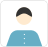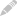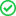Signup as a Tutor

As a tutor you can connect with more than a million students and grow your network.

true
true

# Data Science Using Python Course

No Reviews Yet

Tambaram East, Chennai

Course ID: 52224

You can not pay for your own class.

A2Z Analytics offers Course on Data Science using Python Language. The course will help you master skills, techniques and tools necessary for excelling as a Data Scientist / Machine Learning and Analytics Professional. The course covers the basic concepts like Statistics, Hypothesis testing, Regression Analysis, Prediction, Classification, Clustering, Association Rule Mining and Data Reduction techniques like PCA. The course includes examples and exercises for each of the machine learning algorithms like Naive Bayes, Decision trees, SVM, Apriori Algorithms, Random Forest etc.

The program provides access to high-quality text content content, example repository and other resources that ensure you follow the optimal path to your dream role of data scientist.

Tentative Course Content
Data Science using Python
Module I: Statistics Foundation
1. Basic Concepts in Statistics
Introduction to Statistics, Data Types, Quantitative and Qualitative Data, Types of Variables (Dependent, Independent, Mediating, Moderating), Data Analysis â?? Descriptive, Inferential, Descriptive statistics (Frequencies Distribution, Percentages, Mean, Median, Mode, Standard Deviation, Variance, Standard Variance, Range, Skewness, Kurtosis), Test of Significance, Hypothesis Testing, Null Hypothesis Vs Alternative Hypothesis, Types of Errors, Significance Level (p-value), One-Tailed and Two-Tailed Tests, Reliability and Validity, Exposure to SPSS (IBM PASW 20.0) Environment
2. Exploring Data
Parametric Data â?? Assumptions, Graphing and Screening Data, Working with Groups of Data, Testing for normal distribution, Testing the homogeneity of variance.
3. Inferential Statistics: Comparing Means
t â?? test - One Sample t-test, Independent Samples t-test, Dependent (Paired) Samples t - test, Comparing Means: ANOVA - One-Way, The F-Distribution and F-Ratio, Between-Groups ANOVA, Unplanned and Planned Comparisons, Two-Way Between-Groups ANOVA, MANOVA.
4. Categorical Data Analysis
Chi-Square goodness of Fit Test, Discriminant Analysis
5. Correlation Analysis and Regression Analysis
Bivariate Correlation, Partial Correlation, Introduction to Regression Analysis, Types of Regression Analysis, Simple Linear Regression, Standard Multiple Regression, Method of Least Squares Regression Model, Coefficient of Multiple Determination Regression Model, Standard Error of the Estimate Regression Model, Non-Linear Regression, Non-Linear Regression Models, Algorithms for Complex Non-Linear Models, Hierarchical Regression, Logistic Regression.
6. Data Reduction - Factor Analysis
Exploratory and Confirmatory Factor Analysis (Convergent validity and Discriminant validity), Extraction, Factor Loadings, Rotation, Communalities, Multicollinearity, Eigenvalue and Scree Plot.
7. Non Parametric Data Analysis
Wilcoxon Rank-Sum Test, Mann Whitney Test, Wilcoxon Signed Rank Test, Kruskal-Wallis Test.

Module II: Python Language
1. Python Essentials:
Installation, Python Editors & IDE's, Understand Jupyter notebook, Concept of Packages/Libraries - Important packages(NumPy, SciPy, scikit-learn, Pandas, Matplotlib, statmodels, nltk), Installing & loading Packages & Name Spaces, Data Types & Data objects/structures (strings, Tuples, Lists, Dictionaries), List and Dictionary Comprehensions, Variable & Value Labels â?? Date & Time Values, Basic Operations - Mathematical - string â?? date, Reading and writing data, Simple plotting, Control flow & conditional statements, Debugging & Code profiling, How to create class and modules and how to call them?.
2. Accessing/Importing and Exporting Data using Python modules
Importing Data from various sources, Database Input, â?¢ Viewing Data objects - subsetting, methods, Exporting Data to various formats.
3. Working with Python Packages: Numpy, Pandas, MatPlotLib, Scikit-learn, statmodels, nltk
4. Exploratory Data Analysis (EDA) and Visualization using Python
Introduction exploratory data analysis, Descriptive statistics, Frequency Tables and summarization, Univariate Analysis (Distribution of data & Graphical Analysis), Bivariate Analysis(Cross Tabs, Distributions & Relationships, Graphical Analysis), Creating Graphs- Bar/pie/line chart/histogram/ boxplot/ scatter/ density etc), Important Packages for Exploratory Analysis(NumPy Arrays, Matplotlib, seaborn, Pandas and scipy.stats etc).
5. Data Manipulation, Cleansing, Munging using Python modules
Cleansing Data with Python, Data Manipulation steps(Sorting, filtering, duplicates, merging, appending, sub setting, derived variables, sampling, Data type conversions, renaming, formatting etc), Data manipulation tools(Operators, Functions, Packages, control structures, Loops, arrays etc), Python Built-in Functions (Text, numeric, date, utility functions), Python User Defined Functions, Stripping out extraneous information, Normalizing data, Formatting data, Important Python modules for data manipulation (Pandas, Numpy, re, math, string, datetime etc).

Module III: Machine Learning
1. Introduction to Machine Learning and Predictive Modeling
Introduction, Predictive Modelling, Types of Business problems â?? mapping of techniques, Regression Vs Classification Vs Segmentation Vs Forecasting, Classification System, Major Classes of Learning Algorithms: Supervised Vs Unsupervised Learning, Different Phases of Predictive Modeling (Data Pre-processing, Sampling, Model Building, Validation), Overfitting (Bias-Variance Trade off) & Performance Metrics, Feature engineering & dimension reduction, Concept of optimization & cost function, Concept of gradient descent algorithm, Concept of Cross validation(Bootstrapping, K-Fold validation etc), Model performance metrics (R-square, RMSE, MAPE, AUC, ROC curve, recall, precision, sensitivity, specificity, confusion metrics).

2. Machine Learning Algorithms & Applications â?? Implementation in Python
Linear & Logistic Regression, Segmentation - Cluster Analysis (K-Means), Decision Trees (CART/CD 5.0), Ensemble Learning (Random Forest, Bagging & boosting), Association Rule Mining (Apriori Algorithm), Artificial Neural Networks(ANN), Support Vector Machines(SVM), Other Techniques (KNN, NaÃ¯ve Bayes), Data Reduction (PCA), Introduction to Text Mining using NLTK, Introduction to Time Series Forecasting (Decomposition & ARIMA), Important python modules for Machine Learning (SciKit Learn, stats models, scipy, nltk etc), Fine tuning the models using Hyper parameters, grid search, piping etc.

Copy

Not decided yet.

## Students also enrolled in these CoursesAvg Rating

0 Reviews

2 Students

4 Courses

An experienced trainer and researcher with vast experience in training, teaching, research and data analytics projects.

## Reviews

No reviews currently Be the First to Review

## Discussions### Shan Murali PhD

No Reviews YetStudents Interested 0 (Seats Left 0)

₹ 18,000

Copy

Post your requirement and let us connect you with best possible matches for Data Science Classes Post your requirement now

Enquire

Submit your enquiry for Data Science Using Python Course

Please enter valid question or comment

Connect With A-Z AnalyticsYou have reached a limit!

We only allow 20 Tutor contacts under a category. Please send us an email at support@urbanpro.com for contacting more Tutors.

You Already have an UrbanPro Account

Please Enter valid Email or Phone Number

Forgot Password? Resend OTP OTP Sent

Sorry, we were not able to find a user with that username and password.Thank you for providing more information about your requirement. You will hear back soon from the trainerYou have successfully registeredClass Scheduled at

hour

To join, click

Message copied

How to join a Class?

The UrbanPro Learner app is your study app for this course. You can attend the Demo Class on the app and also get access to the course material, course schedule and ability to communicate with the Tutor. To learn more, watch this video.

Verify you Mobile Number

Please verify your Mobile Number to continue and book a FREE Demo class.UpdatePlease enter 10 digit phone number.

Please Enter a valid Mobile Number

This number is already in use.

Resend